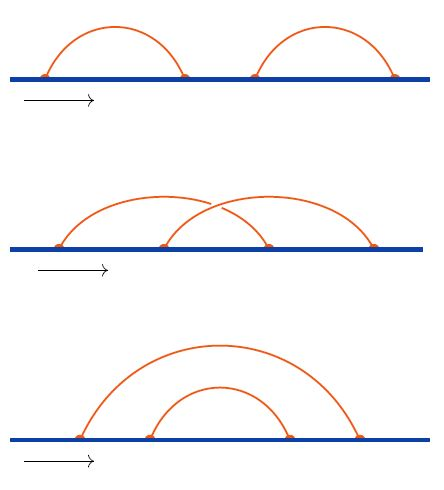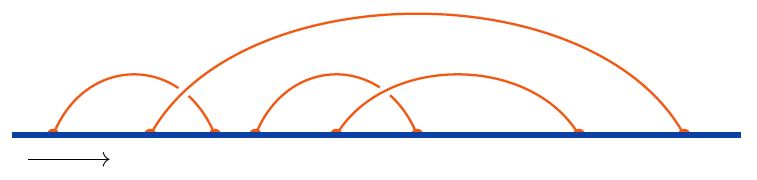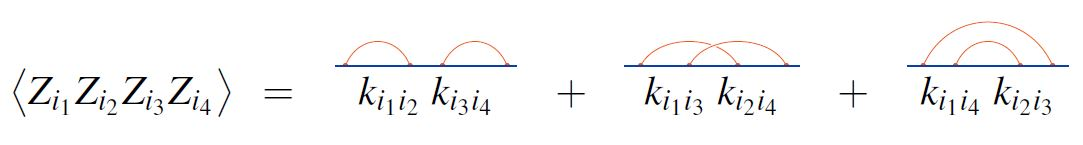# nLab linear chord diagram

Contents

### Context

#### Graph theory

graph theory

graph

category of simple graphs

### Extra structure

#### Knot theory

knot theory

Examples/classes:

Types

knot invariants

Related concepts:

category: knot theory

# Contents

## Idea

A linear chord diagram or arc diagram or rooted chord diagram is a trivalent finite undirected graph with an embedded oriented line and all vertices on that line.

Equivalemtly this is just an n-tuple equipped with a partition into pairs.

The following shows a generic example of a linear chord diagram:The graphics on the right shows all linear chord diagrams with precisely four vertices.

Closing up the line of a linear chord diagram to a circle and remembering the ordering of vertices only op to cyclic permutation, it becomes a round chord diagram, usually just called a chord diagram. Conversely, a linear chord diagram is equivalently a round chord diagram with one of its vertices singled out.

graphics from Sati-Schreiber 19c

## Applications

### Wick’s theorem

The combinatorics of contractions in Wick's theorem is governed by linear chord diagrams:

Let $\{Z_i\}$ be a set of quantum fields/random variables which are free fields/multivariate normally distributed with

$\big\langle Z_i Z_j \big\rangle = k_{i j} \,.$

Then Wick's theorem says that the expectation value of the product of $n \in \mathbb{N}$ of these fields/random variables is the sum over linear chord diagrams with $n$ vertices of the product over the edges $e_i \to e_j$ of the given chord diagram of the factors $k_{i j}$.

For example, for $n = 4$, Wick's theorem says this: